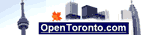# Ounces to Grams Conversion

Enter ouces or grams for conversion:

Select type of conversion:

Rounding options:

One ounce is 28.349 523 grams.

 Ounces to grams Conversion Table: 1 = 28.3 2 = 56.6 5 = 141.7 10 = 283. 20 = 566.9 100 = 2834.9 Grams to Ounces Conversion Table: 1 = 0.035 10 = 0.353 30 = 1.058 60 = 2.116 80 = 2.822 100 = 3.527

One ounce is equal to 437.5 grains. There are 16 avoirdupois ounces in an avoirdupois pound. In 1958 the US and countries of the Commonwealth defined the mass of the international avoirdupois ounce is defined to be equal to 28.349 523 grams. The ounce is commonly used as a unit of mass in the United States and Canada.

Calculators:
MPG calculator
L/100km fuel calculator
L/100km to MPG calculator
MPG to L/100km conversion
Miles to km conversion
mph to kmh conversion
Gallon to Litre Conversion
Pounds to kilograms conversion
Celsius to Fahrenheit
Fahrenheit to Celsius conversion
Recommended Daily Calorie Calculator
BMI Calculator
Ounces to grams conversion
Yards to mm conversion
Yards to meters Conversion
Feet to meters Conversion
Inches to mm Conversion
Time in Hamilton, Ontario